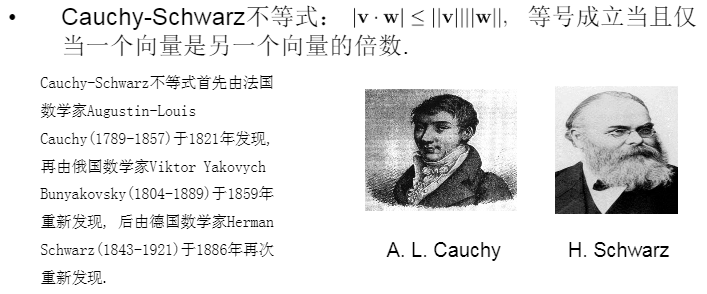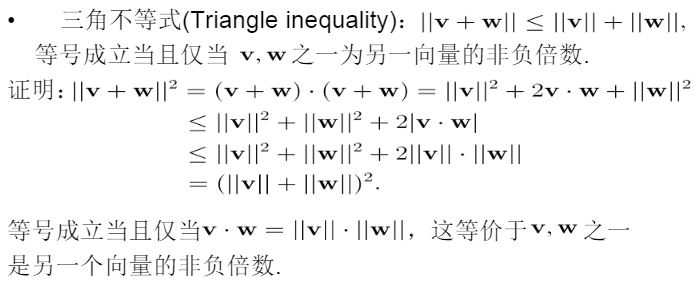Introduction to Linear Algebra 学习笔记(一)

1.向量(Vector)的概念及其基本运算

v=[v1v2]$\begin{bmatrix} v1 \\ v2 \\ \end{bmatrix}$

vector addition：v = [v1v2]$\begin{bmatrix} v1 \\ v2 \\ \end{bmatrix}$w = [w1w2]$\begin{bmatrix} w1 \\ w2 \\ \end{bmatrix}$ 相加得 v + w = [v1+w1v2+w2]$\begin{bmatrix} v1 + w1 \\ v2 + w2 \\ \end{bmatrix}$

scalar multiplication：2v = [2v12v2]$\begin{bmatrix} 2v1 \\2 v2 \\ \end{bmatrix}$ and -v = [v1v2]$\begin{bmatrix} -v1 \\- v2 \\ \end{bmatrix}$

so. 向量的减法是什么样子的就无需多言了~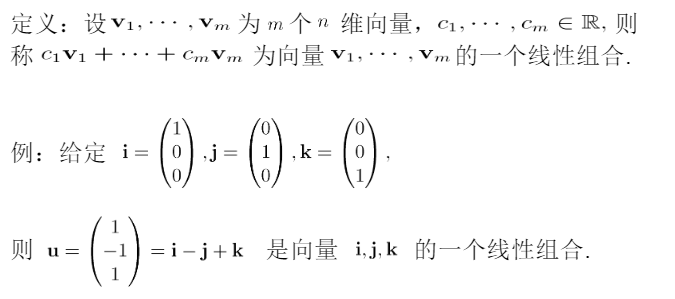tips：zero vector(零向量)

2.向量的可视化（向量的几何意义）

• 两个数字的组合
• 坐标系中以原点(0，0)为起点的箭头（可视化的）
• 在坐标系中单纯戳个看得见的点（emmm……也是可视化的）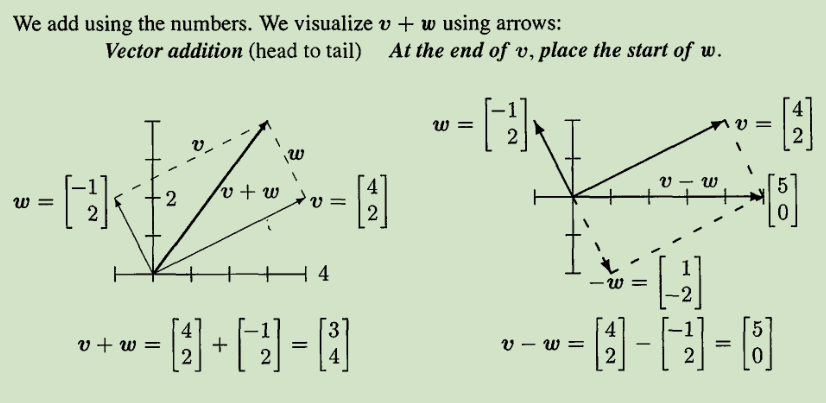ok基础里面最简单的二维向量就先说到这里~二维向量是基础知识里最简单的，然而越简单的东西越重要呢！后面的东西再难都是以这个最简单的东西为基础的，所以没事多拿出这篇笔记翻看下为好！

3.三维空间里的向量

v = 111$\begin{bmatrix} 1 \\ 1 \\-1\\ \end{bmatrix}$w = 234$\begin{bmatrix} 2 \\ 3 \\ 4\\\end{bmatrix}$ 相加得 v + w = 343$\begin{bmatrix} 3 \\4 \\3\\ \end{bmatrix}$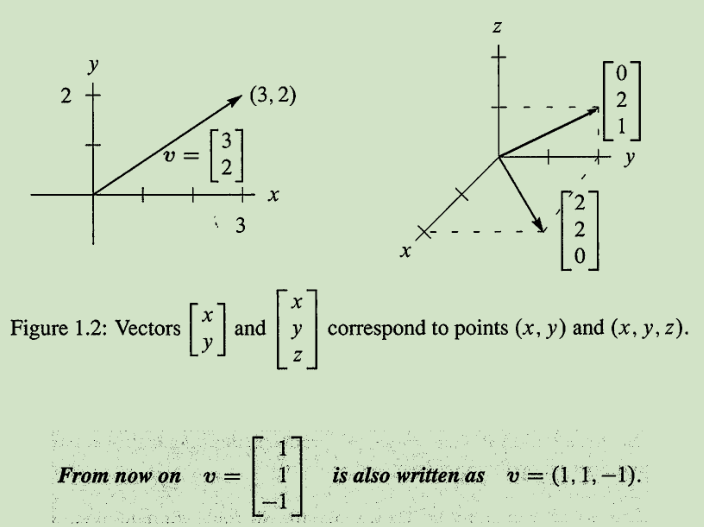1. The combinations c**u** fill a line.
2. The combinations c**u** +d v fill a plane.
3. The combinations c**u** + d v + e**w** fill three-dimensional space.

4.向量的点积（dot product）和长度（length）以及其他

• 向量的点积（dot product）又称为向量的内积（inner product）或数量积（scalar product），下面给出定义：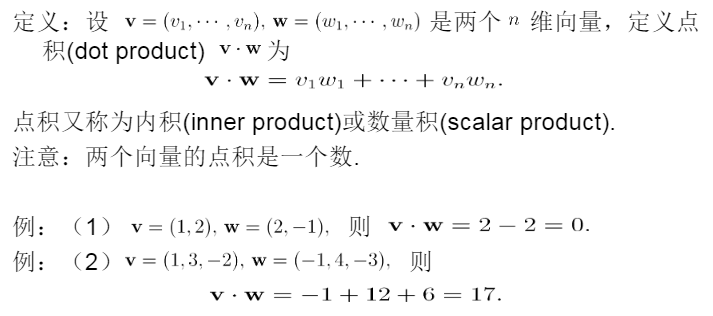• 向量点积的现实意义：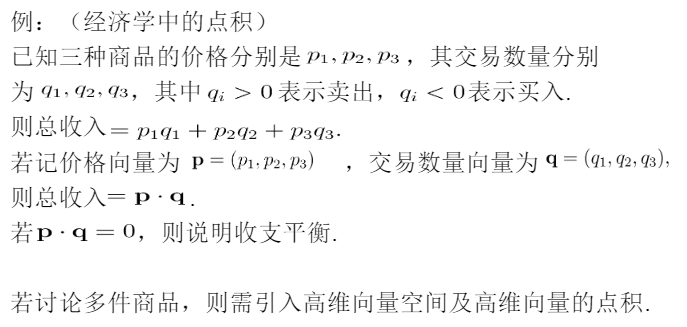• 向量的长度（length）也称模（norm），其定义为：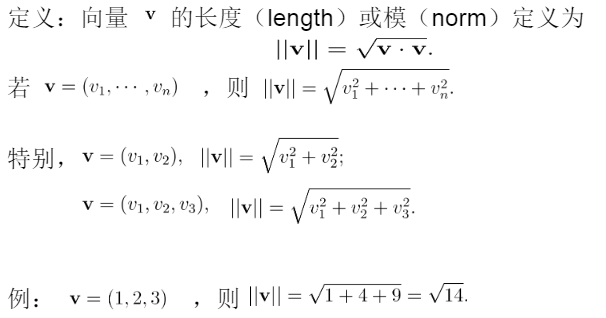• 单位向量（unit vector）和向量点积的性质：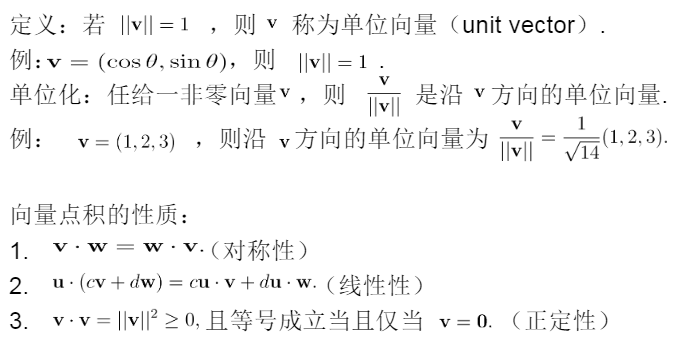• 互相垂直的向量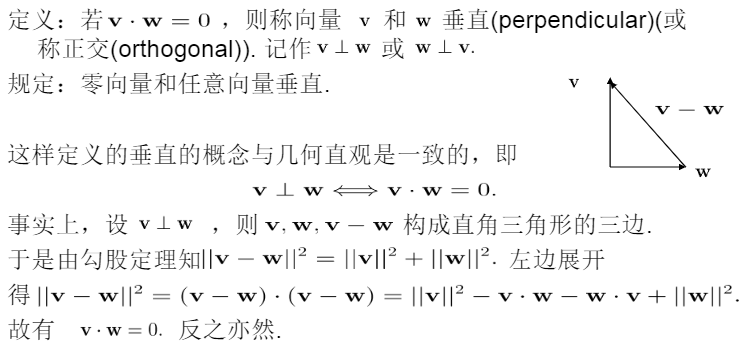• 一个公式，这个公式道出了两个向量的点积和它们长度的关系：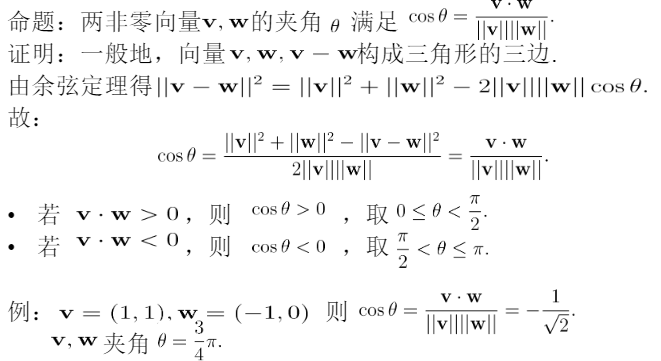• 两个重要的不等式